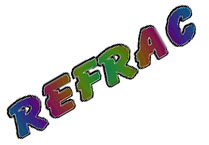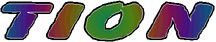That light should reflect off a shiny surface probably comes as no surprise to us. Every child grows up knowing how to use a mirror. That the path of light should change as it crosses from one transparent material to another is not immediately obvious. Light does behave this way and the phenomenon is called refraction. A summary of the rules governing the behavior of light in this context was first suggested by Willibrod Snell who opined that if two transparent materials, such as air and glass, share an interface, the relationship that describes the path of light rays crossing the boundary is given by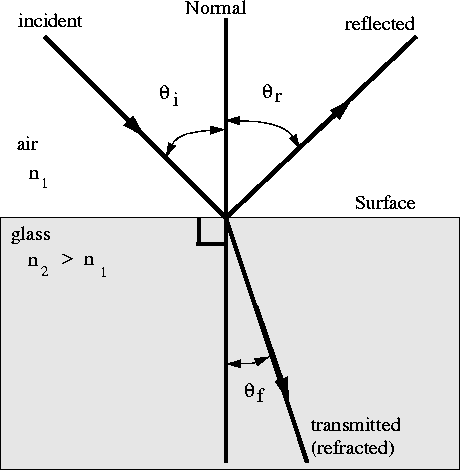For a given pair of transparent materials, as we vary the angle of incidence in one material, the angle of refraction in the other material adjusts itself so that the the ratio of the sines of the angles are the same. sin qA / sin qG = constant = n The in this equation is called the index of refraction, a quantity that relates the bent light path to a significant change in the speed of light as it crosses the boundary. The index compares the speed of light in vacuo to the speed of light is the material in question. By this definition of the index, the value of the index for evacuated space is 1. The index for other common materials is greater than one. The quantity has no units, hence this funny name (not unlike the coefficient of friction we saw earlier).nA sin qA = nB sin qB

http://www.glenbrook.k12.il.us/gbssci/phys/Class/refrn/refrntoc.html
http://www.ps.missouri.edu/rickspage/refract/refraction.html
 Visit HYPERPHYSICS and check out this topic.
Refraction applets

http://www.phy.ntnu.edu.tw/~hwang/fishEye/fishEye.html

http://www.phy.ntnu.edu.tw/java/light/flashLight.html
http://home.a-city.de/walter.fendt/physengl/refraction.htm

\

 Let us apply Snell's law in three situations. Ther first shows a ray in air (n = 1.00) entering glass at an angle of 30 degrees. Now comes a ray initially in glass (n = 1.5) and crossing the boundary into air (n = 1.00) One has to be careful in case #2 that the angle of incidence does not exceed the critical angle.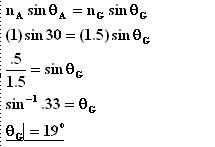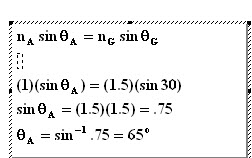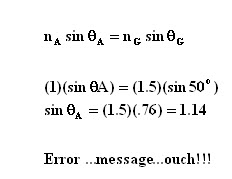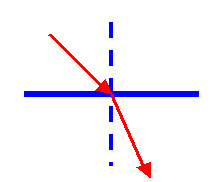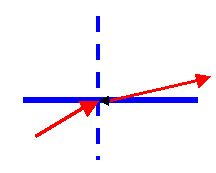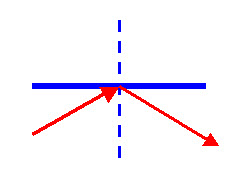The ray of light bends toward the normal. By inspection of the calculation above, if ng > nA, it must be true that theta (air) > theta (glass) Here the light ray bends away from the normal line.By inspection of the calculation above, if ng > nA, it must be true that theta (air) > theta (glass) If the ray is in a higher index material and attempts to cross into the lower index material, it will be reflected rather than refracted.

TOTAL INTERNAL REFLECTION

When light is traveling in a high index material, such as glass, and encounters the interface of a lower index material. such as air (in this context, high and low are relative to each other.) the path of the light changes in such way that the ray bends away from the normal. As Snell's law prescribes, if the angle of incidence in the high index material increases, the angle in the lower index material also increases and, because it is already larger, this angle will get to 90 degrees well before the other angle. The angle in the higher index material which causes the angle in the lower index material to go to 90 degrees is called the critical angle. Use Snell's law to show that the critical angle for an air-glass boundary is 42 degrees if the index for air equals 1 and the index for glass is 1.5.

Should the angle of incidence in the higher index material is greater that the critical angle, the ray of light is reflected back into the high index material, obeying the laws of reflection. This phenomenon has application in fashioning components for periscopes,
binoculars and is at the foundation of a branch of physics dealing with fiber optic cable.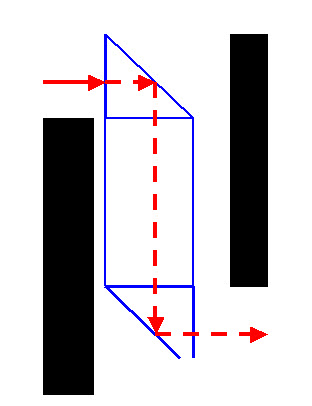A world war II - vintage army tank has heavy armor plate to protect the soldiers inside.. The ttrouble is, how do the soldiers see through the armor plate to drive the tank? The answer is a periscope (from the Greek scope see; peri around..) Consider the situation at left. Thhe black bars represent the armor plate..Light is incident on the vertical face of a prism. (There are no mirrors in this set up.)The angle of incidence is zero; so the angle of rrefraction is also zero.The ray proceeds to a diagonal fface where the angle is 45 deg. Because 45 > 42 (the critical angle for air/glass is 42 degrees). The light continues through the other surfaces and exits at lower right.

 O-07 To witness the effect of total internal reflection, try these applets. http://www.phy.ntnu.edu.tw/~hwang/light/flashLight.htmlhttp://theory.uwinnipeg.ca/physics/java/java/totintrefl/index.html

Why does the distant pavement on a highway appear to be wet?
Go to http://unmuseum.mus.pa.us/mirage.htm

This page was last reviewed by mgosselin 04/04/09